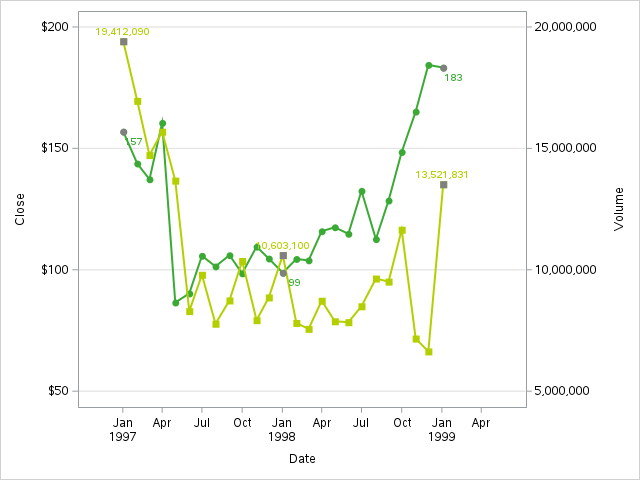## Distinct markers attribute (color) when labeling only certain observations

Hi,

How can I give a distrinct color to only markers where labels selection is displayed ?

data noemers_acute_ZH_YR2;
set noemers_acute_ZH_YR;
LabelVar1 = put(PatientDays,\$NLNUM10.);
if Year not in ("&start_year.", "2019", "2020", "&last_year.") then do;
LabelVar1 = "" ;
LabelVar2 = "" ;
end;
run;

proc sgplot data=noemers_acute_ZH_YR2;
series x=YEAR y=PatientDays / datalabel=LabelVar1 datalabelpos=bottomright markers markerattrs=(color="#3AAA35" symbol=circlefilled ) lineattrs=(color="#3AAA35" thickness=2) /*legendlabel="Standard Lab Value"*/;
series x=YEAR y=Admissions / datalabel=LabelVar2 datalabelpos=bottomleft markers markerattrs=(color="#B4CF00" symbol=squarefilled) lineattrs=(color="#B4CF00" thickness=2) Y2Axis/* legendlabel="New Device"*/;
xaxis label="Year" &styleaxis ;
yaxis label="Patient Days" grid values=(0 to 13000000) ;
y2axis label="Admissions" grid values=(0 to 18000000 ) ;
run;

Many thanks
Laura

5 REPLIES 5

## Re: Distinct markers attribute (color) when labeling only certain observations

@LauraBona92 wrote:

How can I give a distrinct color to only markers where labels selection is displayed ?

Please explain this further. What does "... where labels selection is displayed" mean? If possible, show a fake graph to illustrate what you mean.

--
Paige Miller

## Re: Distinct markers attribute (color) when labeling only certain observations

find hereby the plot generated by my previous code.

I would like grey color markers when label displayed (cfr data step labelvar1 labelvar2 >> when year in (2012, 2019, 2020, 2021)

## Re: Distinct markers attribute (color) when labeling only certain observations

It sounds like you want a group variable for when an observation is marked or not marked. Without data I can't test the code, but try starting with the following and modify it if it isn't exactly correct:

``````data Want;
set noemers_acute_ZH_YR2;
Group1 = (LabelVar1 = "" );
Group2 = (LabelVar2 = "" );
run;

/* UNTESTED */
proc sgplot data=noemers_acute_ZH_YR2;
series x=YEAR y=PatientDays / group=Group1 datalabel=LabelVar1 datalabelpos=bottomright markers markerattrs=(symbol=circlefilled ) lineattrs=(color="#3AAA35" thickness=2) /*legendlabel="Standard Lab Value"*/;
series x=YEAR y=Admissions / group=Group2 datalabel=LabelVar2 datalabelpos=bottomleft markers markerattrs=(symbol=squarefilled) lineattrs=(color="#B4CF00" thickness=2) Y2Axis/* legendlabel="New Device"*/;
xaxis label="Year" &styleaxis ;
yaxis label="Patient Days" grid values=(0 to 13000000) ;
y2axis label="Admissions" grid values=(0 to 18000000 ) ;
run;
``````DanH_sas
SAS Super FREQ

## Re: Distinct markers attribute (color) when labeling only certain observations

For this display, you will need to control the marker color at a group level using the GROUPMC option. To use this option, you will need to create a fake group variable, as @Rick_SAS was showing. Then, you will need a variable to track which observations are labeled. This will be the variable you use on the GROUPMC option. Also, I used a small attributes map to control the color to use for those observations. See the example below to see the general idea. Let me know if you need further explanation.

Thanks!

Dan

``````set sashelp.class (obs=7);
if (name="Alice") then do;
dlab=name;
labeled=1;
end;
else do;
dlab="";
labeled=0;
end;
group=1;
run;

data attrmap;
ID="myid";
value="1";
markercolor="gray";
run;

proc sgplot data=class noautolegend dattrmap=attrmap;
series x=age  y=weight / group=group markers mcattrid=myid
groupmc=labeled datalabel=dlab;
series x=age  y=height / group=group markers mcattrid=myid
groupmc=labeled datalabel=dlab;
run;``````

## Re: Distinct markers attribute (color) when labeling only certain observations

I would create additional 'y' variables in the dataset, and only assign them values for the markers you want to label. And then layer those on top of the lines, with the desired colors (such as gray markers, and the text matching the line color). I think this makes it a little simpler. Here's some sample code, using sample data from sashelp...

``````data noemers_acute_ZH_YR2;
set sashelp.stocks (where=(stock='IBM' and date>='01jan1997'd and date<='31jan1999'd));
if put(date,monname3.) in ('Jan') then do;
close_with_label=close;
LabelVar1 = put(close,\$NLNUM10.);
volume_with_label=volume;
LabelVar2 = put(volume,\$NLNUM10.);
end;
run;

proc sgplot data=noemers_acute_ZH_YR2 noautolegend;
format date year4.;
series x=date y=close / markers markerattrs=(color="#3AAA35" symbol=circlefilled ) lineattrs=(color="#3AAA35" thickness=2);
series x=date y=volume / markers markerattrs=(color="#B4CF00" symbol=squarefilled) lineattrs=(color="#B4CF00" thickness=2) Y2Axis;
series x=date y=close_with_label / markers markerattrs=(color="gray" symbol=circlefilled ) lineattrs=(thickness=0)
datalabel=LabelVar1 datalabelpos=bottom datalabelattrs=(color="#3AAA35");
series x=date y=volume_with_label / markers markerattrs=(color="gray" symbol=squarefilled) lineattrs=(thickness=0) Y2Axis
datalabel=LabelVar2 datalabelpos=top datalabelattrs=(color="#B4CF00");
xaxis label="Date" /*values=('01jan1995'd to '01jan2000'd by year5)*/;
yaxis label="Close" grid values=(50 to 200 by 50);
y2axis label="Volume" grid values=(5000000 to 20000000 by 5000000 );
run;``````Discussion stats
• 5 replies
• 318 views
• 0 likes
• 5 in conversation